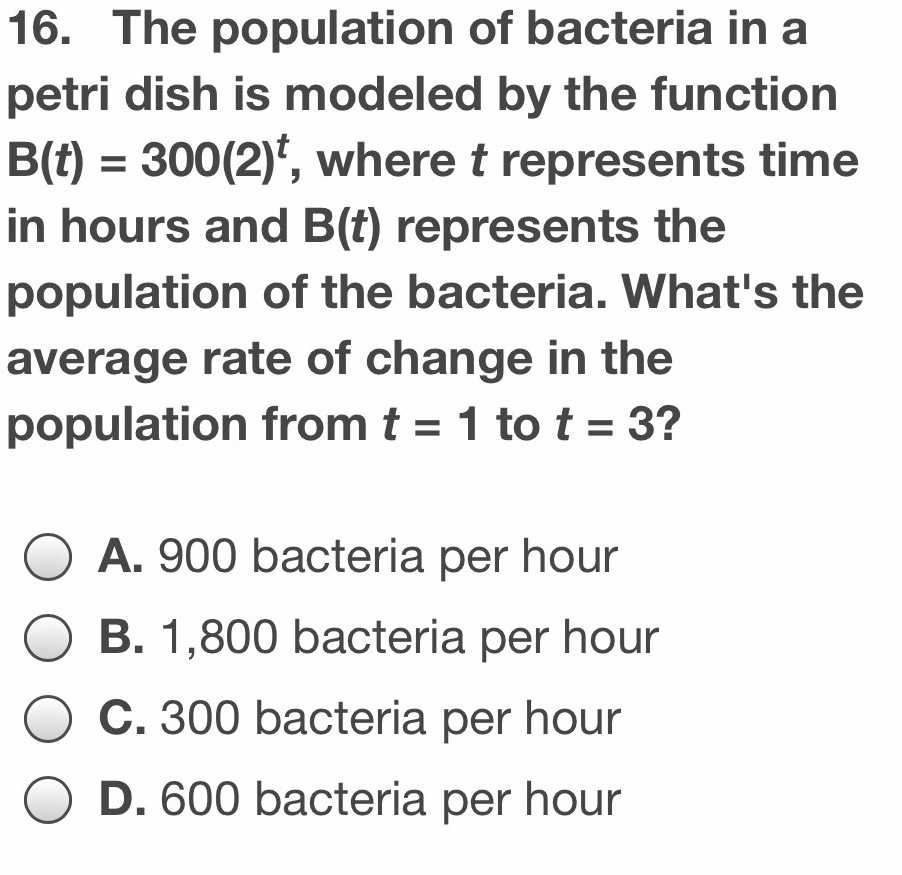### ¿Todavía tienes preguntas de matemáticas?

Pregunte a nuestros tutores expertos
Algebra
Pregunta16. The population of bacteria in a petri dish is modeled by the function $$B ( t ) = 300 ( 2 ) ^ { t } ,$$ where $$t$$ represents time in hours and $$B ( t )$$ represents the population of the bacteria. What's the average rate of change in the population from $$t = 1$$ to $$t = 3$$ ?

A. $$900$$ bacteria per hour

B. $$1,800$$ bacteria per hour

C. $$300$$ bacteria per hour

D. $$600$$ bacteria per hour